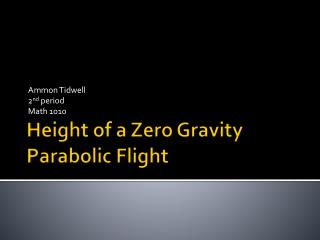Download PresentationHeight of a Zero Gravity Parabolic Flight

Loading in 2 Seconds...

# Height of a Zero Gravity Parabolic Flight - PowerPoint PPT Presentation

Ammon Tidwell 2 nd period Math 1010. Height of a Zero Gravity Parabolic Flight. Height of a Zero-G Flight t Seconds After Starting a Parabolic Flight Path. 3 by 3 system of equations for a, b, and c. 23645=a(2)^2+b(2)+c 4a+2b+c=23645 (1) 32015=a(20)^2+b(20)+c 400a+20b+c=32015 (2)I am the owner, or an agent authorized to act on behalf of the owner, of the copyrighted work described.
Download Presentation## Height of a Zero Gravity Parabolic Flight

An Image/Link below is provided (as is) to download presentation

Download Policy: Content on the Website is provided to you AS IS for your information and personal use and may not be sold / licensed / shared on other websites without getting consent from its author.While downloading, if for some reason you are not able to download a presentation, the publisher may have deleted the file from their server.

- - - - - - - - - - - - - - - - - - - - - - - - - - E N D - - - - - - - - - - - - - - - - - - - - - - - - - -
Presentation Transcript
1. Ammon Tidwell 2nd period Math 1010 Height of a Zero Gravity Parabolic Flight

2. Height of a Zero-G Flight t Seconds After Starting a Parabolic Flight Path

3. 3 by 3 system of equations for a, b, and c • 23645=a(2)^2+b(2)+c • 4a+2b+c=23645 (1) • 32015=a(20)^2+b(20)+c • 400a+20b+c=32015 (2) • 33715=a(40)^2+b(40)+c • 1600a+40b+c=33715 (3)

4. Step 1: Adding to Eliminate • Multiply both sides of equation a by -1 so c can be eliminated when adding equation a and c (-1)4a+2b+c=23645(-1) -4a-2b-c=-23645 (1) 400a+20b+c=32015 (2) 396a+18b =8370 (4)

5. Step 2 • Add to eliminate same variable eliminated on previous slide. Use equation 1 and 3 to eliminate c. -4a-2b-c=-23645 (1) 1600+40b+c=33715 (3) 1596+38b =10070 (5)

6. Step 3: Solve for a • Add equation 4 and 5. Find common multiple to eliminate b by multiplying both sides of the equations by the same number. (-19)396a+18b=8370(-19) (4) -7524a-342b=-159030 (4) (9)1596a+38b=10070(9) (5) 14364a+342b=90630 (5) 6840a= -68400 6840 6840 a=-10

7. Step 4: Solve for b • Now that you know what a equals you can solve for b by plugging the answer for a into equation 4. 396(-10)+18b=8370 (4) -3960+18b=8370 +3960 +3960 18b=12330 18 18 b=685

8. Step 5: Solve for c • Now that you know what a and b equal, you can plug them into the 1st equation and solve for c. 4(-10)+2(685)+c=23645 -40+1370+c=23645 1330+c=23645 -1330 -1330 c=22315

9. Step 6: Form quadratic model • Use the answers previously found for a, b, and c to create a quadratic function. a b c -10x^2+685x+22315

10. Step 7: Find the maximum value of the quadratic function • Find the maximum value of the quadratic function by completing the square. -10x^2=685x+22315 5(-2x^2+137)+22315 Y=5(-2x^2+137x)+22315 -10x^2+685x =-22315 X^2+-1371 * 1 =22315 -137 18769 -10 -10 -10 2 2 10 4 16 X^2-137x+18769=22315+18769 (x-137)^2=41084 2 16 10 10 4 10

11. Graph the parabola • It will be a parabola opening downwards. The maximum value will be 41084 10

12. Reflective Writing • Doing this assignment helped me to realize how math can be used to solve real life problems. Sometimes we think that math, especially difficult problems, is never really used. Doing this assignment gave me an example of how things we learned in class can be used to solve real life problems. I now have a better understanding of how math can be used in life.